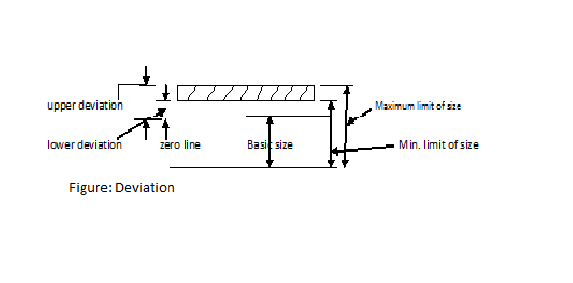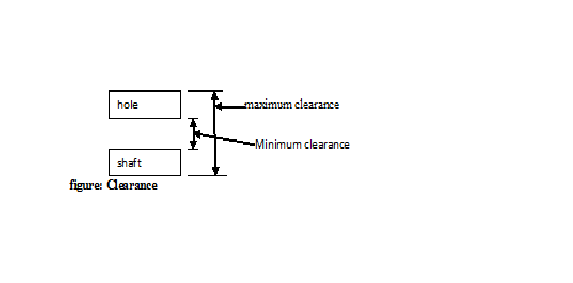Breaking News
Home / Hstudymaterial / JNTUH-HYDERABAD : Machine Drawing Quick Reference Q&A Short Answers

Here are the some of the Machine Drawing Question and Answers. Just Have Glance before going to exam.
Machine Drawing (Mechanical Engineering)

Very-Very important questions to get sure success which covered complete syllabus

Q1: Define basic size?
Ans1: Basic size is that size of a components on which great or less size of a component can be measured.

Q2: Define Limit?
Ans2: It is the higest  and lower limit of a size of a component . The higest size is called high limit of the lowest size is known as low limit.
Q3:Ans3:  Basic size +20mm
Maximum  limit of size =20+0.005 +20.005mm(Max. limit)
Minimum Limit of size =20-0.004=19.996mm(Mini. Limit)

Q4: Define Tolerance?
Ans4: It is the difference between maximum & minimum size of a machine part .Tolerance is the difference between high limit & low limit of a size of a component.

Q5: Calculate the tolerance of the following?
Basic size  =  80+0.002
Ans5:  Tolerance= High limit – Low limit
= 80.002-79.998 = 0.004 mm

Q6: What are the types of tolerance?
Ans6: Tolerances are of two types:-
i)                    Unilateral Tolerance:- This tolerance is given on one side of the basic size.
For example-i)                    Bilateral Tolerance:- In this system the tolerance is given on both the sides of the basic size.
For example-Q7: Define allowance?
Ans7:  The difference between the dimension of two mating ports is called allowance. It is of two types
i)                    Maximum Allowance:- It is the difference between the  maximum size of the hole & minimum size of the shaft.
ii)                  Minimum Allowance:-  It is the difference between the  maximum size of the shaft & minimum size of the hole.

Q8: Given, i) The maximum hole size =  30.099 mm and minimum shaft size = 30.050 mm. calculate the maximum allowance?
(ii). The maximum hole size =  30.075 mm and minimum shaft size = 30.060 mm. calculate the minimum allowance?
Ans8:  i)  Maximum allowance = 30.099 – 30.050 = 0.049 mm
ii). Minimum Allowance = 30.075 – 30.060 = 0.015 mm

Q9: Define FIT.
Ans9: The assembly of two mating parts is called Fit.

Q10: Write different type of Fits?
Ans10: 1) Clearance Fit:- In clearance fit , shaft is smaller than hole. This type of fits gives loose fitting. There must be some degree of freedom between a shaft & a hole.
2) Interference Fit:- In interference Fit, there is always a negative allowance between the largest hole size & the smallest shaft size. In this fit, the shaft is always larger than the hole.
3) Transition Fit:- In this type of Fits, the fit may be either clearance fit or interference fit. Any pair of parts mating with transition fit may fit with interference while on other pair with the same fit have a clearance fit.

Q11: Define Deviation.
Ans11: It is defined as the difference between actual size or Limit sizes, either maximum or minimum, and the corresponding basic size.

Q12:Define actual deviation?
Ans12: It is the algebraic difference between the actual measured size & corresponding basic size.

Q13: Define upper deviation?
Ans13:  The algebraic difference between the maximum limit of a size & the corresponding basic size.
Q14: Define lower deviation?
Ans14:  The algebraic difference between the minimum limit of a size & the corresponding basic size.
figure:deviationQ15: Define zero line.
Ans15: Zero line is the line of zero deviation and represents the basic size.

Q16: Define tolerance Zone.
Ans16: Tolerance Zone is the algebraic difference between maximum limit of size & minimum limit of size.In other words, tolerance zone is the algebraic difference between upper deviation & lower deviation.

Q17: Define functional Deviation.
Ans17: Functional deviation is the upper or lower deviation which is closest to basic size.

Q18: Define hole basis system.
Ans18: It is the standard system of  limits & fits. The size of the hole is kept constant & the size of the shaft is kept variable to get the different types of fits is known as hole basis system. The symbol ‘H’ is a fundamental deviation is selected for the holes, when the hole basis system is follows.Q19: Define shaft basis system.
Ans 19: When the sizes of the shaft is kept sonstant in a standard system of limits & fits, and variations given to the hole for obtaining different types of fits, is known as shaft basis system. The fundamental deviation symbol ‘H’ is selected for shaft basis.

Q20: Define fundamental tolerance .
Ans20: This is also known as grade of Tolerance in Indian Standard System, there are 18 grade of tolerances represented by number symbols both for hole and shaft such as ITOI, ITO, IT1 to IT16. A high number gives a large tolerance zone . fundamental tolerance are indicated for sizes ranging upto 525 mm.

Q21: Define Limit System?
Ans21: The system in which deviations are accepted are called limit system.

Q22: Define standardization.
Ans22:  Standardisation means the size of a machine element accepted nationally or internationally.

Q23: What do you mean by H7/g6?
Ans23: It is sliding fit. Its application are in clutches & shafts.

Q24: What do you mean by φ25 H8 u7?
Ans24:  Basic size = 25 mm
H8 means an h hole to tolerance grade 8.
u7 means a u shaft to tolerance grade 7.

Q25: Define clearance.
Ans25: It is defined as the difference between the dimensions of  the hole & the shaft assigned intentionally to obtain a particular type of fit. It may be positive or negative when the shaft size is smaller than the hole size, it will be positive & when the shaft size is bigger than the hole , it will be negative.
Maximum Clearance:- It is the difference between maximum hole & minimum shaft.
Minimum clearance:- In clearance fit, the minimum clearance is the difference between the minimum hole & the maximum shaft.
figure: ClearanceQ  26:Define Interferance.
Ans26: The  negative difference between the size of the hole & shaft before its assembly in made.
Q27: If the size of the hole is 40.02,  40.00 and the size of shaft is 39.96,  39.94 . Find (i) Hole tolerance   (ii) shaft tolerance   (iii) allowance?

Ans27:  (i) Hole tolerance = Maximum limit – Minimum limit
= 40.02 – 40.00 = 0.02 mm
(ii) shaft tolerance =  max. limit – min.limit= 39.96 – 39.94 = 0.02 mm
(iii) Allowance = min. size of hole – max size of shaft = 40.00 – 39.96 = 0.04 mm

Q28: Define surface roughness.
Ans28:  Surface roughness may be defined as the fine irregularities in the surface texture.

Q29: Define Roughness height?
Ans29: It is also known as roughness average (Ra) is an arithmetical average of the roughness deviation.

Q30: Define roughness width.
Ans30: It is the distance parallel to the nominal surface between the two successive peaks, which constitute the predominant pattern of the roughness. It is measured in millimeter.